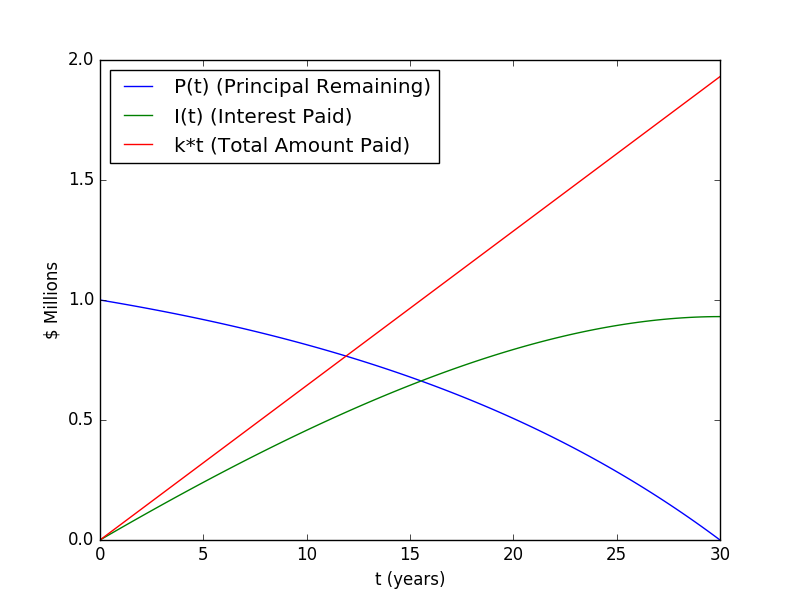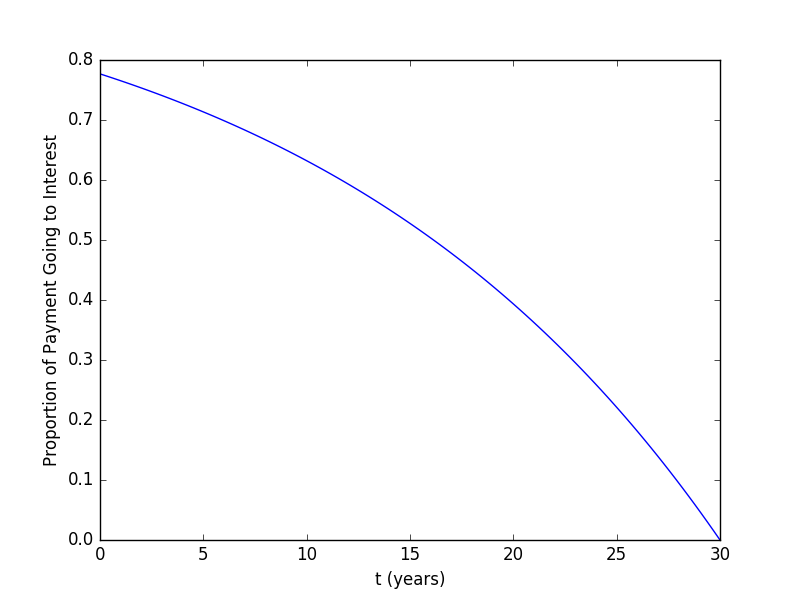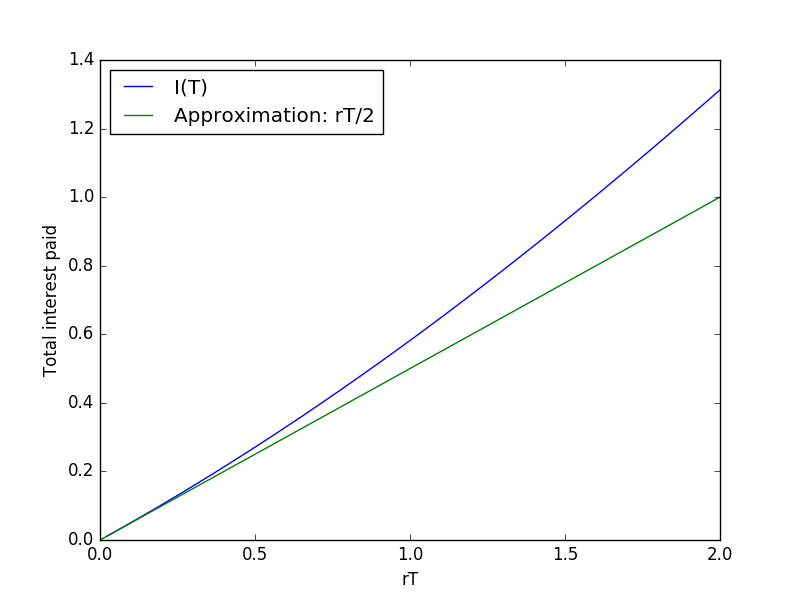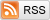# Continuous Time Lending

January 15, 2017

Assume a borrower takes out an installment loan of size $1$ and makes continuous-time payments on it. The installment loan starts at time $0$, ends at time $T$, and has an interest rate of $r$, compounding continually. We'll let $P(t)$ be the principal owed by the borrower at time $t$, and $I(t)$ be the interest they've paid on the loan so far. Unlike in discrete time lending, we don't need to keep track of the amount of unpaid interest owed by the borrower; it's always zero since the borrower makes continuous payments. To check your comprehension, $P(0) = 1$, $P(T) = 0$, $I(0) = 0$, and $I(T)$ is the total amount of interest the borrower will pay on their loan (assuming no defaults, fees, or prepayment).

The first thing to do is figure out the borrower's payments and the resulting values of $P(t)$ and $I(t)$. Since this is an installment loan with equal sized payments, in each infinitesimal interval $dt$ the borrower makes payments $kdt$, where $k$ is some constant that we'll determine shortly. So over the course of the loan the borrower pays a total of $kT$. The amount of principal the borrower has paid at time $t$ is $1 - P(t)$ and the amount of interest they've paid is $I(t)$, so the total amount they've paid at time $t$ is:

$$\boxed{ kt = 1 - P(t) + I(t) }$$

This is the "account balancing" equation.

Now, in each infinitesimal interval $dt$, the borrower accrues and pays interest of $rP(t)dt$, so we have:

$$\boxed{ \frac{dI(t)}{dt} = rP(t) }$$

This is the "interest accrual" equation.

The preceding two equations define a differential equation for $P(t)$ that we can solve. Solving the first equation for $I(t) = kt + P(t) - 1$ and substituting into the second equation gives us:

$$k + \frac{dP(t)}{dt} = rP(t)$$

The solution to this kind of differential equation has the form $P(t) = A - Be^{rt}$. Plugging this into the differential equation immediately gives us $k = Ar$. We can then solve for $A$ and $B$ using the two boundary conditions

\begin{align} 1 & = P(0) = A - B \\ 0 & = P(T) = A - Be^{rT} \end{align}

which gives us:

$$A = \frac{e^{rT}}{e^{rT} - 1}\\ B = \frac{1}{e^{rT} - 1}$$

So finally, we have:

$$\boxed{ k = r\frac{e^{rT}}{e^{rT} - 1} \\ P(t) = \frac{e^{rT} - e^{rt}}{e^{rT} - 1} \\ I(t) = \frac{1 - e^{rt} + rte^{rT}}{e^{rT} - 1} }$$

But enough math for now, time for a plot! Below we plot the continuous-time approximation of a 30 year, one million dollar fixed-rate mortgage at 5.000%. Plugging and chugging, we get that $\frac{k}{12}$ is \$5,363, and that$I(30)$is \$930,825. (So the mortgage will cost about \$1.93M in total, with monthly payments of \$5,363).One other interesting quantity is $\frac{I'(t)}{k}$, the proportion of the payment that goes to interest. With a bit of math, we see that this quantity is:

$$\frac{I'(t)}{k} = 1 - \frac{e^{rt}}{e^{rT}}$$

Plotted for our 5.000%, 30 year mortgage:One thing you may have noticed about all of these lending equations is that they are all in terms of $rt$ and $rT$. In other words, they never depend on time directly, only through time multiplied by $r$. So, for example, a 30 year mortgage at 5% is in a certain sense equivalent to a 15 year mortgage at 10%, with time running twice as slow. (Both will cost \$1.93M in total, the 15-year mortgage will have twice the monthly payments as the 30-year mortgage, and the principal remaining and interest paid for the 15 year mortgage at time$t$will be the same for the 30 year mortgage at time$2t$). This observation is especially interesting when it comes to$I(T)$, the total amount paid in interest. It's easy to see that this is: $$I(T) = \frac{rTe^{rT}}{e^{rT} - 1} - 1$$ A Taylor expansion at$rT=0$shows that this is approximately$\frac{rT}{2}$for small values of$rT$, and you can understand why: For small values of$rT$, most of the borrowers payments go to principal reduction, so the average value of$P(t)$is about$\frac{1}{2}$. On average, then, the borrower pays$r\frac{1}{2}$in interest each year for$T$years, giving us the approximation. Plotting$I(T)$as a function of$rT$shows that this approximation works reasonably well up to about$T = 0.5\$:For the interested, the code generating these plots can be found here.